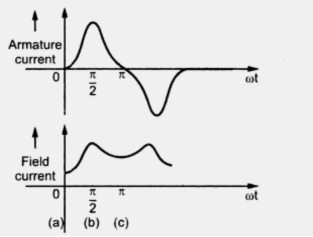Short Circuit Transients for Synchronous Generator Part2

1.3 Short Circuit Phenomenon
Consider a two pole elementary single phase alternator with concentrated stator winding as shown in Fig. 4.Fig. 4

The corresponding waveforms for stator and rotor currents are shown in the Fig. 5.Fig. 2

Let short circuit occurs at position of rotor shown in Fig. 4(a) when there are no stator linkages. After 1/4 Rev as shown Fig. 4(b), it tends to establish full normal linkage in stator winding. The stator opposes this by a current in the shown direction as to force the flux in the leakage path. The rotor current must increase to maintain its flux constant. It reduces to normal at position (c) where stator current is again reduces to zero. The waveform of stator current and field current shown in the Fig. 5. changes totally if the position of rotor at the instant of short circuit is different. Thus the short circuit current is a function of of relative position of stator and rotor.
Using the theorem of constant linkages a three phase short circuit can also be studied. After the instant of short circuit the flux linking with the stator will not change. A stationary image of main pole flux is produced in the stator. Thus a d.c. component of current is carried by each phase. The magnitude of d.c. component of current is different for each phase as the instant on the voltage wave at which short circuit occurs is different for each phase. The rotor tries to maintain its own poles. The rotor current is normal each time when rotor poles occupy the position same as that during short circuit and the current in the stator will be zero if the machine is previously unloaded. After one half cycle from this position the stator and rotor poles are again coincident but the poles are opposite. To maintain the flux linkages constant, the current in rotor reaches to its peak value.
The stationary field produced by poles on the stator induces a normal frequency emf in the rotor. Thus the rotor current is fluctuating whose resultant a.c. component develops fundamental frequency flux which rotates and again produces in the stator winding double frequency or second harmonic currents. Thus the waveform of transient current consists of fundamental, a.c. and second harmonic components of currents.
Thus whenever short circuit occurs in three phase generator then the stator currents are distorted from pure sine wave and are similar to those obtained when an alternating voltage is suddenly applied to series R-L circuit.
1.4 Stator Currents during Short Circuit
If a generator having negligible resistance, excited and running on no load is suddenly undergoing short circuit at its terminals, then the emf induced in the stator winding is used to circulate short circuit current through it. Initially the reactance to be taken into consideration is not the synchronous reactance of the machine. The effect of armature flux (reaction) is to reduce the main field flux. But the flux linking with stator and rotor can not change instantaneously because of the induction associated with the windings. Thus at the short circuit instant, the armature reaction is ineffective. It will not reduce the main flux. Thus the synchronous reactance will not come into picture at the moment of short circuit. The only limiting factor for short circuit current at this instant is the leakage reactance.
After some time from the instant of short circuit, the armature reaction slowly shows its effect and the alternator then reaches to steady state. Thus the short circuit current reaches to high value for some time and then settles to steady value.
It can be seen that during the initial instant of short circuit is dependent on induced emf and leakage reactance which is similar to the case which we have considered previously of voltage source suddenly applied to series R-L circuit. The instant in the cycle at which short occurs also affects the short circuit current. Near zero e.m.f. (or voltage) it has doubling effect. The expressions that we have derived are applicable only during initial conditions of short circuit as the induced emf also reduces after some tome because of increased armature reaction.
The short circuit currents in the three phases during short circuit are as shown in the Fig. 6Fig. 6 Short circuit current waveforms in the three phases of alternator